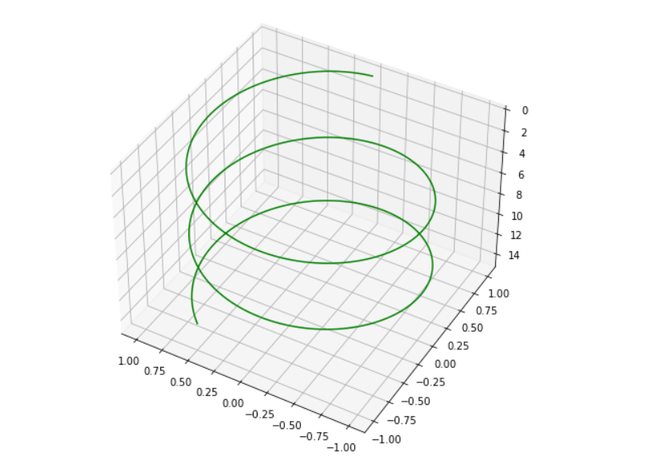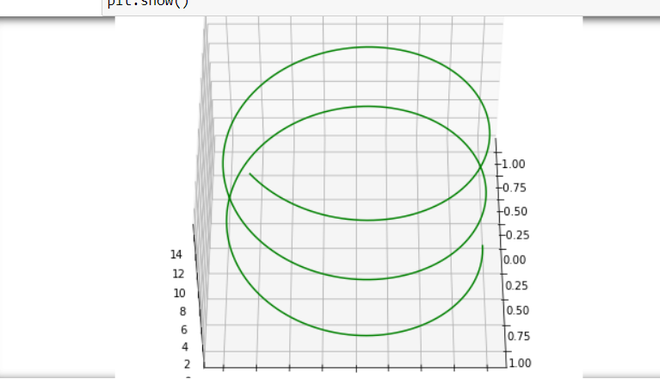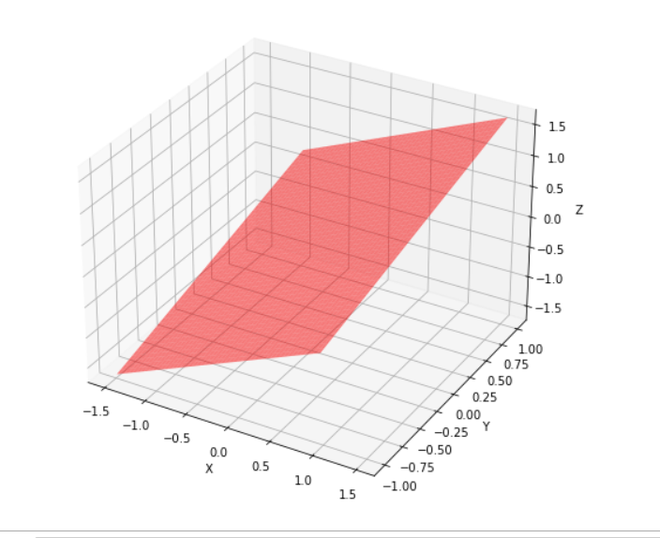GeeksforGeeks App
Open AppBrowser
Continue

# How to change angle of 3D plot in Python?

Prerequisites: Matplotlib, NumPy

In this article, we will see how can we can view our graph from different angles, Here we use three different methods to plot our graph. Before starting let’s see some basic concepts of the required module for this objective.

• Matplotlib is a multi-platform data visualization library built on NumPy arrays and designed to work with the broader SciPy stack
• Numpy is a general-purpose array-processing package. It provides a high-performance multidimensional array object, and tools for working with these arrays. It is the fundamental package for scientific computing with Python
• mpl_toolkits provides some basic 3D plotting (scatter, surf, line, mesh) tools.

Approach:

• Import required library.
• Create a figure.
• Create a datasheet.
• Change angle of the 3D plot
• Show Graph.

Step 1: Import libraries.

## Python3

 `from` `mpl_toolkits ``import` `mplot3d``import` `numpy as np``import` `matplotlib.pyplot as plt`

Step 2: Plotting 3-D axis figure.

## Python3

 `from` `mpl_toolkits ``import` `mplot3d``import` `numpy as np``import` `matplotlib.pyplot as plt`` ` `fig ``=` `plt.figure(figsize ``=` `(``8``, ``8``))``ax ``=` `plt.axes(projection ``=` `'3d'``)`

Step 3: Creating a Datasheet for all the 3-axis of the sample.

## Python3

 `from` `mpl_toolkits ``import` `mplot3d``import` `numpy as np``import` `matplotlib.pyplot as plt`` ` `fig ``=` `plt.figure(figsize ``=` `(``8``, ``8``))``ax ``=` `plt.axes(projection ``=` `'3d'``)`` ` `# Data for a three-dimensional line``z ``=` `np.linspace(``0``, ``15``, ``1000``)``x ``=` `np.sin(z)``y ``=` `np.cos(z)``ax.plot3D(x, y, z, ``'green'``)`

Step 4: Use view_init() can be used to rotate the axes programmatically.

Syntax: view_init(elev, azim)

Parameters:

• ‘elev’ stores the elevation angle in the z plane.
• ‘azim’ stores the azimuth angle in the x,y plane.D constructor.

## Python3

 `from` `mpl_toolkits ``import` `mplot3d``import` `numpy as np``import` `matplotlib.pyplot as plt`` ` `fig ``=` `plt.figure(figsize ``=` `(``8``,``8``))``ax ``=` `plt.axes(projection ``=` `'3d'``)`` ` `# Data for a three-dimensional line``z ``=` `np.linspace(``0``, ``15``, ``1000``)``x ``=` `np.sin(z)``y ``=` `np.cos(z)``ax.plot3D(x, y, z, ``'green'``)`` ` `ax.view_init(``-``140``, ``60``)`

Below is the full Implementation:

Example 1: In this example, we will plot a curve graph with an elevated angle of -140 degrees and a horizontal angle of 60 degrees.

## Python3

 `from` `mpl_toolkits ``import` `mplot3d``import` `numpy as np``import` `matplotlib.pyplot as plt`` ` `fig ``=` `plt.figure(figsize ``=` `(``8``,``8``))``ax ``=` `plt.axes(projection ``=` `'3d'``)`` ` `# Data for a three-dimensional line``z ``=` `np.linspace(``0``, ``15``, ``1000``)``x ``=` `np.sin(z)``y ``=` `np.cos(z)``ax.plot3D(x, y, z, ``'green'``)`` ` `ax.view_init(``-``140``, ``60``)`` ` `plt.show()`

Output:Example 2: In this example, we will plot a curve graph with an elevated angle of 120 degrees and a horizontal angle of 30 degrees.

## Python3

 `from` `mpl_toolkits ``import` `mplot3d``import` `numpy as np``import` `matplotlib.pyplot as plt`` ` `fig ``=` `plt.figure(figsize ``=` `(``8``, ``8``))``ax ``=` `plt.axes(projection ``=` `'3d'``)`` ` `# Data for a three-dimensional line``z ``=` `np.linspace(``0``, ``15``, ``1000``)``x ``=` `np.sin(z)``y ``=` `np.cos(z)``ax.plot3D(x, y, z, ``'green'``)`` ` `ax.view_init(``120``, ``30``)`` ` `plt.show()`

Output:Example 3: In this example, we will plot a rectangular plate graph with an angle view of 50 degrees.

## Python3

 `import` `numpy as np``from` `matplotlib ``import` `pyplot as plt``from` `mpl_toolkits.mplot3d ``import` `Axes3D``from` `math ``import` `sin, cos`` ` `fig ``=` `plt.figure(figsize ``=` `(``8``,``8``))``ax ``=` `fig.add_subplot(``111``, projection ``=` `'3d'``)`` ` `#creating Datasheet``y ``=` `np.linspace(``-``1``, ``1``, ``200``)``x ``=` `np.linspace(``-``1``, ``1``, ``200``)``x,y ``=` `np.meshgrid(x, y)`` ` `#set z values``z ``=` `x ``+` `y `` ` `# rotate the samples by changing the value of 'a'``a ``=` `50` ` ` `t ``=` `np.transpose(np.array([x, y, z]), ( ``1``, ``2``, ``0``))`` ` `m ``=` `[[cos(a), ``0``, sin(a)],[``0``, ``1``, ``0``],``     ``[``-``sin(a), ``0``, cos(a)]]`` ` `X,Y,Z ``=` `np.transpose(np.dot(t, m), (``2``, ``0``, ``1``))`` ` `#label axes``ax.set_xlabel(``'X'``)``ax.set_ylabel(``'Y'``)``ax.set_zlabel(``'Z'``)`` ` `#plot figure``ax.plot_surface(X,Y,Z, alpha ``=` `0.5``,``                ``color ``=` `'red'``)`` ` `plt.show()`

Output:My Personal Notes arrow_drop_up